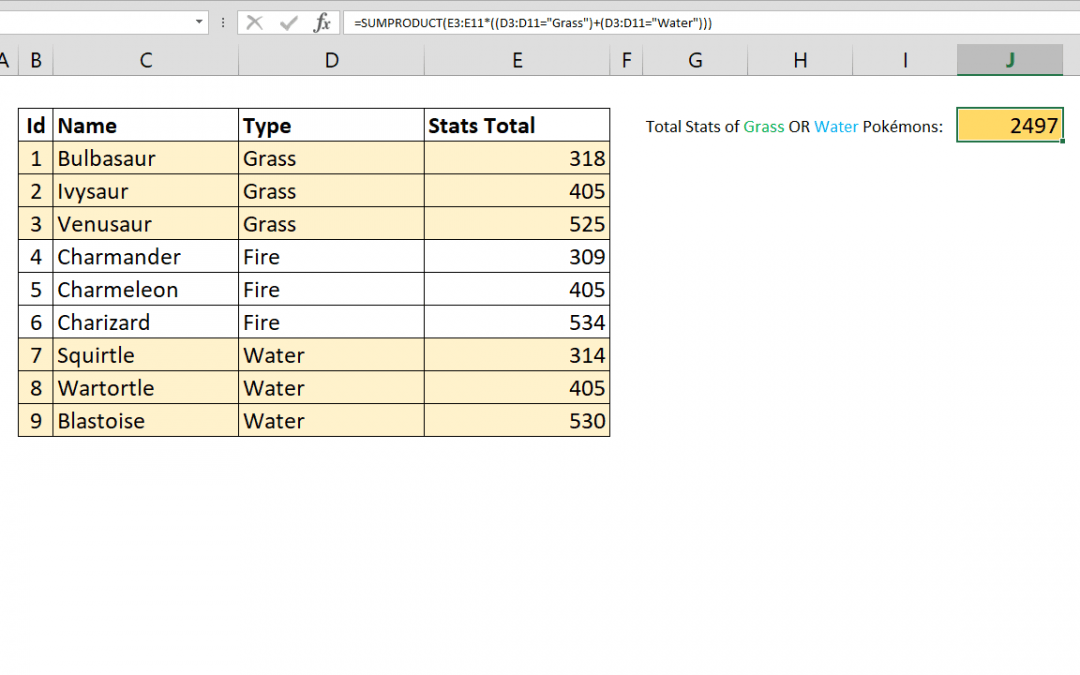Select Page## How to sum values with OR operator using SUMPRODUCT with multiple criteria

The SUMIFS function can sum values based on multiple conditions. However, these conditions are all linked with AND operator. As a result, all conditions should be fulfilled to return a value. This article shows How you can sum values with multiple OR operators using...## How to SUM 2d ranges with SUMPRODUCT

Summing values by a condition may sound easy. If not, please see Crunch Data Even Faster with the SUMIFS Function article as well. You can easily sum values by condition(s) with SUMIF and SUMIFS functions; with a limitation. The sum range and criteria ranges should...## How to do a cell count if not equal to a variable or even an error code

You can use the COUNTIFS function to count the values in ranges that meet certain criteria. The criteria can contain a not equal logic as well. However, the COUNTIFS function requires individual arguments for each range-criteria pair. This requirement can make it...## How to count if not equal to x or y in Excel

The COUNTIFS function can count the values in multiple ranges with multiple criteria. The function counts each given range-criteria pair and combines the result with AND logical operator. Unfortunately, there is no quick and easy way to use an OR logic with the...## How to make Excel calculate faster

Excel is a fairly an efficient software given that it can perform complex calculations. However, depending on your system, it can also get pretty slow when running complicated calculations. How formulas are used, and size of the workbook are typically the culprit if...## Control Shift Enter Excel Shortcut (CSE) and Array Formulas

Control Shift Enter Excel shortcut, as known as CSE, is a key combination you can use to apply array functions.CSE abbreviation is also used for array formulas that are entered using the Control + Shift + Enter key combination when entering a formula. Array formulas...